# Overview: SEQDESIGN Procedure

The purpose of the SEQDESIGN procedure is to design interim analyses for clinical trials. Clinical trials are experiments on human subjects to demonstrate the efficacy and safety of new drugs or treatments. A simple example is a trial to test the effectiveness of a new drug in humans by comparing the outcomes in a group of patients who receive the new drug with the outcomes in a comparable group of patients who receive a placebo.

A clinical trial is conducted according to a plan called a protocol. A protocol details the objectives of the trial, the data collection process, and the analysis. The protocol specifies the null hypothesis and an alternative hypothesis, a test statistic, the probabilityof a Type I error, the probabilityof a Type II error, the sample size needed to attain a specified power of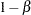at an alternative reference, and critical values that are associated with the test statistic.

In a fixed-sample trial, data about all individuals are first collected and then examined at the end of the study. Most major trials have committees that periodically monitor safety and efficacy data during the trial and recommend that a trial be stopped for safety concerns such as an unacceptable toxicity level. In certain situations, the committee might recommend that a trial be stopped for efficacy. In contrast to a fixed-sample trial, a group sequential trial provides for interim analyses before the completion of the trial while maintaining the specified overall Type I and Type II error probabilities.

A group sequential trial is most useful in situations where it is important to monitor the trial to prevent unnecessary exposure of patients to an unsafe new drug, or alternatively to a placebo treatment if the new drug shows significant improvement. In most cases, if a group sequential trial stops early for safety concerns, fewer patients are exposed to the new treatment than in the fixed-sample trial. If a trial stops early for efficacy reasons, the new treatment is available sooner than it would be in a fixed-sample trial. Early stopping can also save time and resources.

A group sequential design provides detailed specifications for a group sequential trial. In addition to the usual specification for a fixed-sample design, it provides the total number of stages (the number of interim stages plus a final stage) and a stopping criterion to reject, to accept, or to either reject or accept the null hypothesis at each interim stage. It also provides critical values and the sample size at each stage for the trial.

At each interim stage, the data collected at the current stage in addition to the data collected at previous stages are analyzed, and statistics such as a maximum likelihood test statistic and its associated standard error are computed. The test statistic is then compared with critical values that are generated from the sequential design, and the trial is stopped or continued. If a trial continues to the final stage, the null hypothesis is either rejected or accepted. The critical values for each stage are chosen in such a way that the overallandare maintained at the specified levels.

Figure 80.1 shows a two-sided symmetric group sequential trial that stops early to reject the null hypothesis that the parameter Trt is zero.

Figure 80.1 Sequential Plot for Two-Sided Test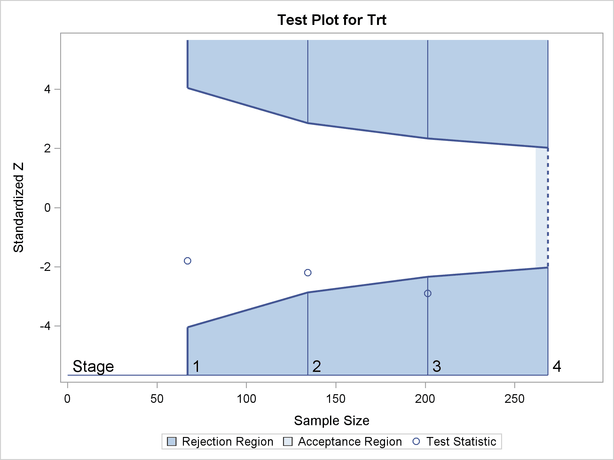The trial has four stages, which are indicated by the vertical lines labeled,,, and. With early stopping to reject the null hypothesis, the lower rejection boundary is constructed by connecting the lower critical values for the stages. Similarly, the upper rejection boundary is constructed by connecting the upper critical values for the stages. The horizontal axis indicates the sample size for the group sequential trial, and the vertical axis indicates the values of the test statistic on the standardized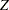scale.

At each interim stage, if the test statistic falls into a rejection region (the darker shaded areas in Figure 80.1), the trial stops and the null hypothesis is rejected. Otherwise, the trial continues to the next stage. At the final stage (stage), the null hypothesis is rejected iffalls into a rejection region. Otherwise, the null hypothesis is not rejected. In Figure 80.1, the test statistic does not fall into the rejection regions for stagesand, and so the trial continues to stage. At stage, the test statistic falls into the rejection region, and the null hypothesis is rejected.

A group sequential trial usually involves six steps:

1. You specify the statistical details of the design, including the null and alternative hypotheses, a test statistic for the hypothesis test, the Type I and II error probabilities, a stopping criterion, the total number of stages, and the relative information level at each stage.

2. You compute the boundary values for the trial based on the specifications in Step 1. You also compute the sample size required at each stage for the specified hypothesis test.

3. At each stage, you collect additional data with the required sample sizes. The data available at each stage include the data collected at previous stages in addition to the data collected at the current stage.

4. At each stage, you analyze the available data with a procedure such as the REG procedure, and you compute the test statistic.

5. At each stage, you compare the test statistic with the corresponding boundary values. You stop the trial to reject or accept the hypothesis, or you continue the trial to the next stage. If you continue the trial to the final stage, you either accept or reject the hypothesis.

6. After the trial stops, you compute parameter estimates, confidence limits for the parameter, and a-value for the hypothesis test.

You use the SEQDESIGN procedure at Stepto compute the initial boundary values and required sample sizes for the trial. You use the companion SEQTEST procedure at Stepto compare the test statistic with its boundary values. At stage, the boundary values are derived by using the boundary information tables created by the SEQDESIGN procedure. These boundary information tables are structured for input to the SEQTEST procedure. At each subsequent stage, the boundary values are derived by using the test information tables are created by the SEQTEST procedure at the previous stage. These test information tables are also structured for input to the SEQTEST procedure. You also use the SEQTEST procedure at Stepto compute parameter estimates, confidence limits, and-values after the trial stops.

The flowchart in Figure 80.2 summarizes the steps in a typical group sequential trial and the relevant SAS procedures.

Figure 80.2 Group Sequential Trial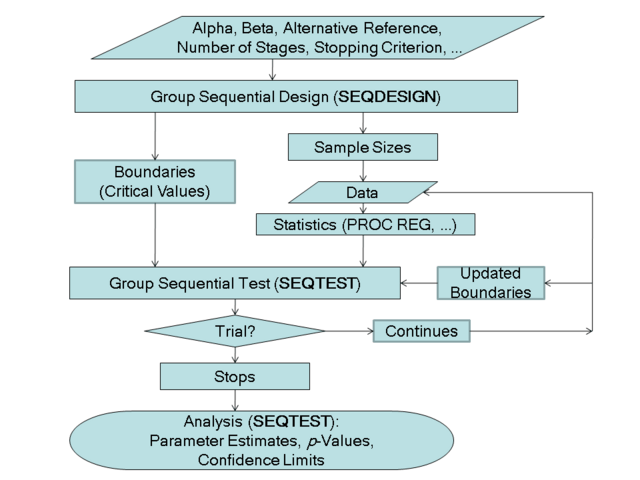### Features of the SEQDESIGN Procedure

The SEQDESIGN procedure assumes that the standardizedtest statistics for the null hypothesis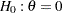at the stages have the joint canonical distribution with the information levels at the stages for the parameter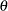. This implies that these test statistics are normally distributed. If the test statistic is not normally distributed, then it is assumed that the test statistic is computed from a large sample such that the statistic has an approximately normal distribution. See the section Statistical Assumptions for Group Sequential Designs for a detailed description of the joint canonical distribution.

You can use the SEQDESIGN procedure to compute required sample sizes for commonly used hypothesis tests. Note that for a fixed-sample design, you should use the POWER and GLMPOWER procedures to compute sample sizes.

The applicable tests include tests for binomial proportions and the log-rank test for two survival distributions. See the section Applicable One-Sample Tests and Sample Size Computation, the section Applicable Two-Sample Tests and Sample Size Computation, and the section Applicable Regression Parameter Tests and Sample Size Computation for examples of applicable tests in group sequential trials.

At each stage, the data are analyzed with a statistical procedure such as the REG procedure, and a test statistic and its associated information level are computed. The information level is the amount of information available about the unknown parameter. For a maximum likelihood statistic, the information level is the inverse of its variance.

At each stage, you use the SEQTEST procedure to derive the boundary values that correspond to the information level associated with the test statistic. You then use the SEQTEST procedure to compare the test statistic with these boundary values. When a trial is stopped at an interim stage or at the final stage, the SEQTEST procedure also derives parameter estimates, confidence limits for the parameter, and a-value for hypothesis testing.

### Output from the SEQDESIGN Procedure

In addition to computing the boundary values for a group sequential design, the SEQDESIGN procedure computes the following quantities:

• maximum sample size (as a percentage of the corresponding fixed-sample size) if the trial does not stop at an interim stage

• average sample numbers (as percentages of the corresponding fixed-sample sizes for nonsurvival data or fixed-sample numbers of events for survival data) under various hypothetical references, including the null and alternative references

• stopping probabilities at each stage under various hypothetical references to indicate how likely it is that the trial will stop at that stage

• sample sizes required at each stage for the specified hypothesis test

• numbers of events required at each stage for the specified hypothesis test with survival data

You can create more than one design with multiple DESIGN statements in the SEQDESIGN procedure and then choose the design with the most desirable features. The next two subsections introduce some basic aspects of group sequential designs that are useful for getting started with the SEQDESIGN procedure.